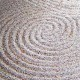## Welcome to the Treehouse Community

The Treehouse Community is a meeting place for developers, designers, and programmers of all backgrounds and skill levels to get support. Collaborate here on code errors or bugs that you need feedback on, or asking for an extra set of eyes on your latest project. Join thousands of Treehouse students and alumni in the community today. (Note: Only Treehouse students can comment or ask questions, but non-students are welcome to browse our conversations.)

### Looking to learn something new?

Treehouse offers a seven day free trial for new students. Get access to thousands of hours of content and a supportive community. Start your free trial today.# Hi, I entered in my code an assignment that works (I checked it in the console), but it won't pass the check.

var chance = Math.round(Math.random(0,20))

index.html
```<!DOCTYPE html>
<html lang="en">
<title>JavaScript Foundations: The Math Object</title>
<script>
var diameter = 5.75,
a = 17,
b = 42,
c = 1337,
ageIfILiveToYear2100 = (new Date(2100, 0, 1) - new Date(1995, 5, 16, 7, 20)) / (1000 * 60 * 60 * 24 * 365.242);

/*
The JavaScript Math object has the following
constant (among others):
Math.PI
Approximately 3.14159. Pi (π) is used
to determine the circumference of a circle
given its diameter by multiplying the diameter
by π. Geometery refresher: the circumference
of a circle is the distance around the outside
edge. The diameter is the width of the circle.

The JavaScript Math object also has the following
methods (among others):
Math.random()
Returns a random number between 0 and 1
Math.max(a, b, ...)
Returns the largest value of the passed
arguments
Math.floor(a)
Returns the value of a number rounded down to
the nearest integer
*/

var circumference = diameter * Math.PI;
var chance = Math.round(Math.random(0, 20) * 10);

</script>
<body>
<h1>JavaScript Foundations</h1>
<h2>The Math Object</h2>
<script src="viewer.js"></script>
</body>
</html>
```PLUS

Paul,

try it this way, remove the parentheses in your last statement. If you remove it, the equation should be good

var chance = Math.random()*20+1;

see if this works for you.PLUS

Not a problem... If you have any questions, please feel free to ask away. You can follow on facebook where I will start putting up little tips and tricks very shortly... Have a great day =)